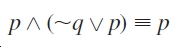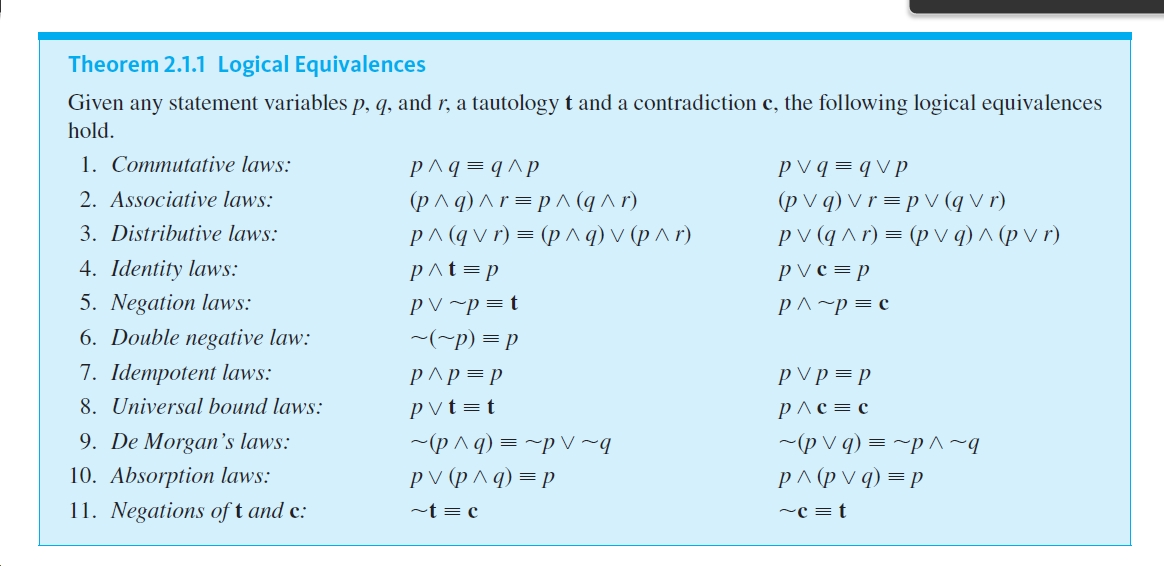# ^b-p^(~qv p) =P Theorem 2.1.1 Logical EquivalencesGiven any statement variables p, q, and r, a tautology t and a contradiction c, the following logical equivalenceshold.1. Commutative laws:P Vq = qVp(p V q) V r = p V (q V r)p V (q ^ r) = (p v q) ^ (p v r)P^q = q^p(p^q)^r=p ^ (q ^ r)рл (qvr) %3D(рлд)v(р^г)2. Associative laws:3. Distributive laws:4. Identity laws:p^t=pV c = pp5. Negation laws:PV ~p=tP^~p = c6. Double negative law:~(~p) = p7. Idempotent laws:p V p = pP^p=p8. Universal bound laws:Pvt=tрлс3Dс9. De Morgan's laws:~(p ^ q) = ~p V ~qp V (p ^ q) = P(p V q) = ~p^~qp^ (p v q) = p10. Absorption laws:11. Negations of t and c:~t = c~c = t

Question
6 views

Use Theorem 2.1.1 to verify the logical equivalences. Supply a reason for each step.help_outlineImage Transcriptionclose^b- p^(~qv p) =P fullscreenhelp_outlineImage TranscriptioncloseTheorem 2.1.1 Logical Equivalences Given any statement variables p, q, and r, a tautology t and a contradiction c, the following logical equivalences hold. 1. Commutative laws: P Vq = qVp (p V q) V r = p V (q V r) p V (q ^ r) = (p v q) ^ (p v r) P^q = q^p (p^q)^r=p ^ (q ^ r) рл (qvr) %3D(рлд)v(р^г) 2. Associative laws: 3. Distributive laws: 4. Identity laws: p^t=p V c = p p 5. Negation laws: PV ~p=t P^~p = c 6. Double negative law: ~(~p) = p 7. Idempotent laws: p V p = p P^p=p 8. Universal bound laws: Pvt=t рлс3Dс 9. De Morgan's laws: ~(p ^ q) = ~p V ~q p V (p ^ q) = P (p V q) = ~p^~q p^ (p v q) = p 10. Absorption laws: 11. Negations of t and c: ~t = c ~c = t fullscreen
check_circle

Step 1

Given:

...

### Want to see the full answer?

See Solution

#### Want to see this answer and more?

Solutions are written by subject experts who are available 24/7. Questions are typically answered within 1 hour.*

See Solution
*Response times may vary by subject and question.
Tagged in

### Math